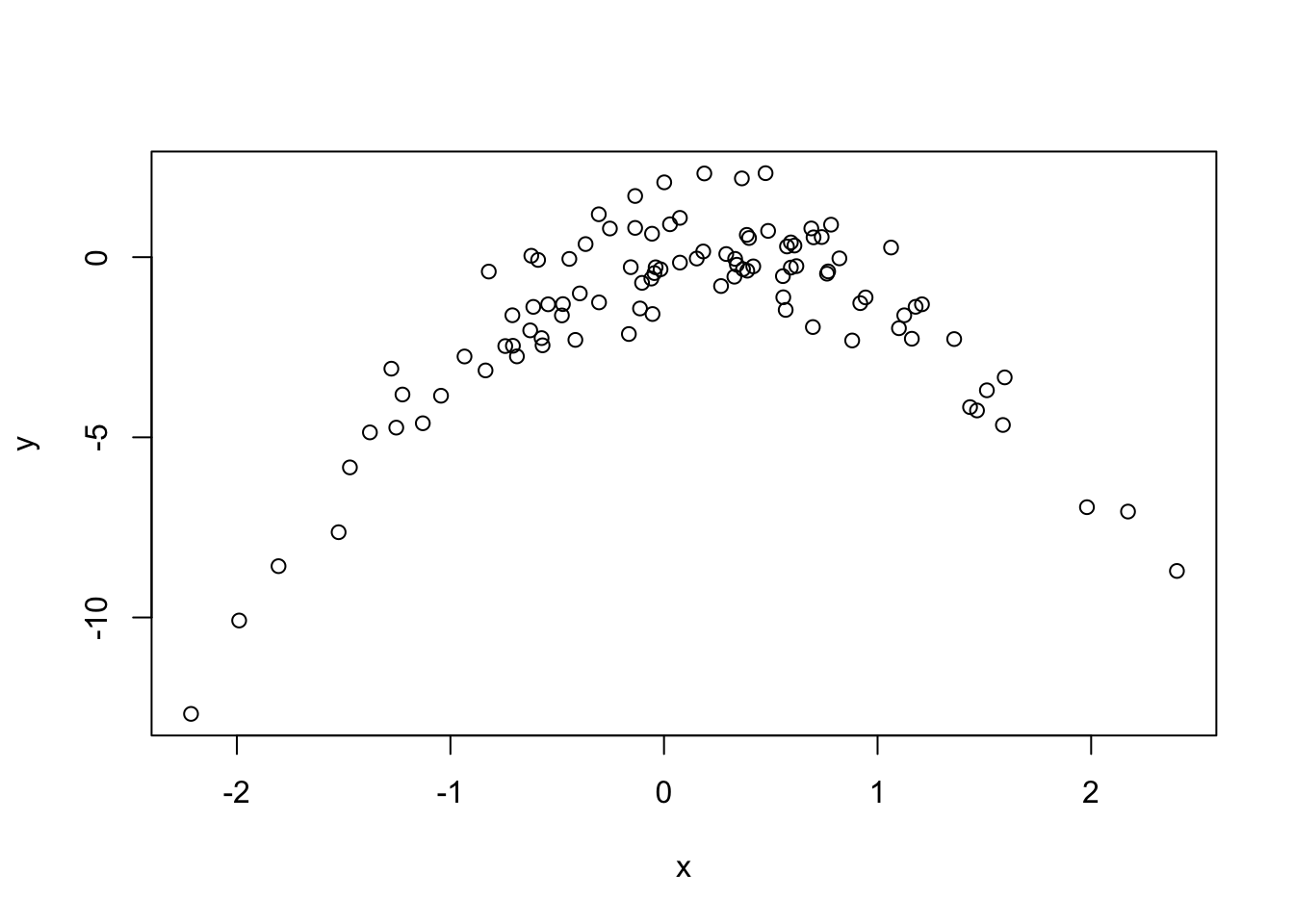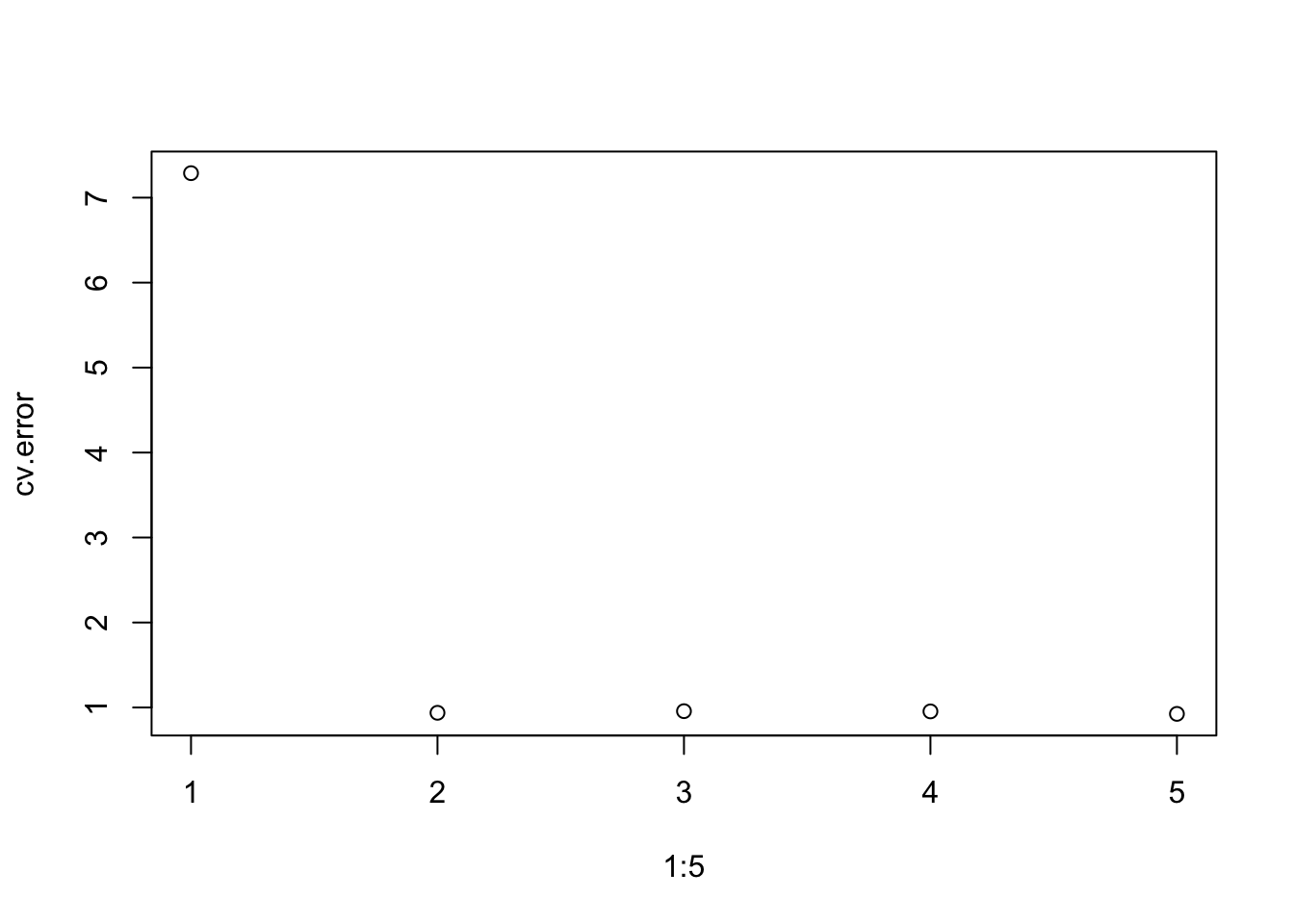ISLR Home

# Question

p200

We will now perform cross-validation on a simulated data set. (a) Generate a simulated data set as follows:

> set.seed(1)
> x=rnorm(100)
> y=x-2*x^2+rnorm(100)

In this data set, what is n and what is p? Write out the model used to generate the data in equation form.

1. Create a scatterplot of X against Y . Comment on what you find.

2. Set a random seed, and then compute the LOOCV errors that result from fitting the following four models using least squares:

1. Y = β0 + β1X + ε

$$Y=\beta_0 + \beta_1 X + \epsilon$$

1. Y = β0 + β1X + β2X2 + ε

$$Y=\beta_0 + \beta_1 X + \beta_2 X2 + \epsilon$$

1. Y = β0 +β1X +β2X2 +β3X3 +ε

$$Y=\beta_0 + \beta_1 X + \beta_2 X2 + \beta_3 X3 + \epsilon$$

1. Y = β0 +β1X +β2X2 +β3X3 +β4X4 +ε.

$$Y=\beta_0 + \beta_1 X + \beta_2 X2 + \beta_3 X3 + \beta_4 X4 + \epsilon$$

Note you may find it helpful to use the data.frame() function to create a single data set containing both X and Y .

1. Repeat (c) using another random seed, and report your results. Are your results the same as what you got in (c)? Why?

2. Which of the models in (c) had the smallest LOOCV error? Is this what you expected? Explain your answer.

3. Comment on the statistical significance of the coefficient esti- mates that results from fitting each of the models in (c) using least squares. Do these results agree with the conclusions drawn based on the cross-validation results?

library(ISLR)

# 8a

set.seed(1)
x=rnorm(100)
y=x - 2*x^2 + rnorm(100)
# n = 100
# p = 2

# Y = b0 + b1x1 + ...+ bpXp 

# 8b Plot

plot(x,y)# 8c

library(boot) # cv.glm()

# More on poly()
# https://stackoverflow.com/questions/19484053/what-does-the-r-function-poly-really-do

loocv.fn = function(df, exponent) {
glm.fit = glm(y~poly(x, exponent), df)
}

set.seed(1)
df = data.frame(x,y)

cv.error=rep(0,5) # 5 zeros
#(0,0,0,0,0)

for (i in 1:5) {
print(i)
glm.fit=glm(y~poly(x, i))
print(cv.glm(df, glm.fit)$delta) # cv.error[i] = cv.glm(df, glm.fit)$delta[]
}
##  1
##  7.288162 7.284744
##  2
##  0.9374236 0.9371789
##  3
##  0.9566218 0.9562538
##  4
##  0.9539049 0.9534453
##  5
##  0.9247836 0.9243911
#cv.error

# 8d

set.seed(10)
cv.error=rep(0,5) # 5 zeros

for (i in 1:5) {
print(i)
glm.fit=glm(y~poly(x, i))
#print(cv.glm(df, glm.fit)$delta) cv.error[i] = cv.glm(df, glm.fit)$delta
}
##  1
##  2
##  3
##  4
##  5
plot(1:5, cv.error)We get the same answer because LOOCV only leaves out one data point out Cycles through all points one at a time to train.

# 8e

Because original equation was quadratic, the quadratic fit will match the best

# 8f

summary(glm.fit)
##
## Call:
## glm(formula = y ~ poly(x, i))
##
## Deviance Residuals:
##     Min       1Q   Median       3Q      Max
## -1.9699  -0.6020  -0.1543   0.5656   2.1218
##
## Coefficients:
##             Estimate Std. Error t value Pr(>|t|)
## (Intercept)  -1.5500     0.0952 -16.282  < 2e-16 ***
## poly(x, i)1   6.1888     0.9520   6.501  3.8e-09 ***
## poly(x, i)2 -23.9483     0.9520 -25.156  < 2e-16 ***
## poly(x, i)3   0.2641     0.9520   0.277    0.782
## poly(x, i)4   1.2571     0.9520   1.321    0.190
## poly(x, i)5   1.4802     0.9520   1.555    0.123
## ---
## Signif. codes:  0 '***' 0.001 '**' 0.01 '*' 0.05 '.' 0.1 ' ' 1
##
## (Dispersion parameter for gaussian family taken to be 0.9062565)
##
##     Null deviance: 700.852  on 99  degrees of freedom
## Residual deviance:  85.188  on 94  degrees of freedom
## AIC: 281.76
##
## Number of Fisher Scoring iterations: 2

The linear and quadratic have significance p-value < 0.05